Tamilnadu State Board New Syllabus Samacheer Kalvi 8th Maths Guide Pdf Chapter 2 Measurements Ex 2.2 Text Book Back Questions and Answers, Notes.

## Tamilnadu Samacheer Kalvi 8th Maths Solutions Chapter 2 Measurements Ex 2.2

Question 1.
Find the perimeter and area of the figures given below. (π = $$\frac{22}{7}$$)
(i)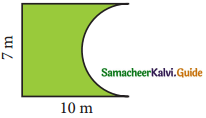Length of the arc of the semicircle = $$\frac{1}{2}$$ × 2πr units
= $$\frac{22}{7} \times \frac{7}{2}$$ m =11 m
∴ Perimeter = Sum of all lengths of sides that form the closed boundary
P = 11 + 10 + 7 + 10 m
Perimeter = 38 m
Area = Area of the rectangle – Area of semicircle
= (l × b) – $$\frac{1}{2}$$ πr2 sq. units= 50.75 m2 (approx)
Area of the figure = 50.75 m2 approx.(ii)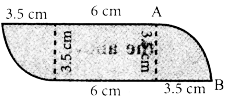Perimeter = sum of outside lengths
Length of the arc of quadrant circle = $$\frac{1}{4}$$ × 2πr units
= $$\frac{1}{2} \times \frac{22}{7}$$ × 3.5cm
= 11 × 0.5 cm = 5.5cm
∴ Length of arc of 2 sectors 2 × 5.5 cm
= 11 cm
∴ Perimeter P = 11 + 6 + 3.5 + 6 + 35 cm
P = 30 cm
Area Area of 2 quadrant circle + Area of a rectangle.
= 2 × $$\frac{1}{4}$$πr2 + lb sq. units
= ($$\frac{1}{2} \times \frac{22}{7}$$ × 3.5 × 3.5) + (6 × 3.5) cm2
= (11 × 3.5 × 0.5) + 21 cm2
= (19.25 + 21) cm2 = 40.25 cm2
∴ Area = 40.25 cm2 approxQuestion 2.
Find the area of the shaded part in the following figures. (π = 3.14)
(i)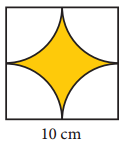Area of the shaded part = Area of 4 quadrant circles of radius $$\frac { 10 }{ 2 }$$ cm
= 4 × $$\frac { 1 }{ 4 }$$ × πr2 = 3.14 × $$\frac { 10 }{ 2 }$$ × $$\frac { 10 }{ 2 }$$ cm2
= $$\frac { 314 }{ 4 }$$ cm2 = 78.5 cm2
Area of the shaded part = 78.5 cm2
Area of the unshaded part = Area of the square – Area of shaded part
= a2 – 78.5 cm2 = (10 × 10) – 78.5 cm2
= 100 – 78.5cm2 = 21.5cm2
Area of the unshaded part = 21.5 cm2 (approximately)

(ii)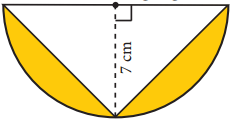Area of the shaded part = Area of semicircle – Area of the triangle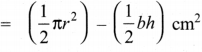= $$\frac { 1 }{ 2 }$$ × 3.14 × 7 × 7 – $$\frac { 1 }{ 2 }$$ × 14 × 7cm2
= $$\frac{153.86}{2}$$ – 49 cm2 = 76.93 – 49 cm2
= 27.3 cm2
∴ Area of the shaded part = 27.93 cm2 (approximately)Question 3.
Find the area of the combined figure given which is got by joining of two parallelograms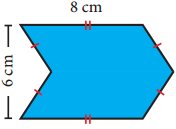Area of the figure = Area of 2 parallelograms with base 8 cm and height 3 cm
= 2 × (bh) sq. units
= 2 × 8 × 3cm2 = 48 cm2
∴ Area of the given figure = 48 cm2

Question 4.
Find the area of the combined figure given, formed by joining a semicircle of diameter 6 cm with a triangle of base 6 cm and height 9 cm. (π = 3.14)Area of the figure = Area of the semicircle of radius 3 cm + 2 (Area of triangle with b = 9 cm and h = 3 cm)
= $$\left(\frac{1}{2} \pi r^{2}\right)+\left(2 \times \frac{1}{2} b h\right)$$ sq. units
= $$\frac { 1 }{ 2 }$$ × 3.14 × 3 × 3 + (2 × $$\frac { 1 }{ 2 }$$ × 9 × 3)cm2
= $$\frac{28.26}{2}$$ + 27 cm2 = 14.13 + 27 cm2 = 41.13 cm2
∴ Area of the figure = 41.13 cm2 (approximately)Question 5.
The door mat which is in a hexagonal shape has the following measures as given in the figure. Find its area.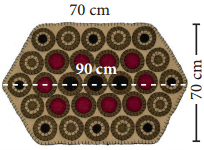Area of the doormat = Area of 2 trapezium
Height of the trapezium h =$$\frac { 70 }{ 2 }$$ cm: a = 90 cm; b = 70 cm
∴ Area of the trapezium = $$\frac { 1 }{ 2 }$$ h (a + b) sq. units
Area of the door mat = 2 × $$\frac { 1 }{ 2 }$$ × 35 (90 + 70)cm2
= 35 × 160 cm2 = 5600 cm2
∴ Area of the door mat = 5600 cm2

Question 6.
A rocket drawing has the measures as given in the figure. Find its area.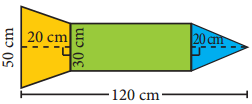Area = Area of a rectangle + Area of a triangle + Area of a trapezium
For rectangle length l = 120 – 20 – 20 cm = 80 cm
For the triangle base = 30 cm
Height = 20 cm
For the trapezium height h = 20 cm
Parallel sided a = 50 cm
b = 30cm
∴ Area of the figure (l × b) + ($$\frac { 1 }{ 2 }$$ × base × height) + $$\frac { 1 }{ 2 }$$ × h × (a + b)sq. units
= (80 × 30) + ($$\frac { 1 }{ 2 }$$ × 30 × 20) + $$\frac { 1 }{ 2 }$$ × 20 × (50 + 30) cm2
= 2400 + 300 + 800 cm2 = 3500 cm2
Area of the figure = 3500 cm2Question 7.
Find the area of the irregular polygon shaped fields given below.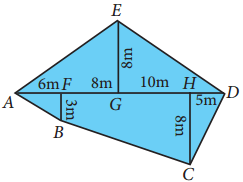Area of the triangle = $$\frac { 1 }{ 2 }$$ bhsq.units
Area of the trapezium = $$\frac { 1 }{ 2 }$$ × h × (a + b) sq.units
Area of the trapezium FBCH = $$\frac { 1 }{ 2 }$$ × (10 + 8) × (8 + 3)m2 = 9 × 11 = 99 m2 ….. (1)
Area of the ∆DHC = $$\frac { 1 }{ 2 }$$ × 8 × 5 m2 = 20 m2 ….. (2)
Area of ∆EGD = $$\frac { 1 }{ 2 }$$ × 8 × 15m2 = 60 m2 …….. (3)
Area of ∆EGA = $$\frac { 1 }{ 2 }$$ × 8 × (8 + 6)m2 = 4 × 14 m2
Area of ∆BFA = $$\frac { 1 }{ 2 }$$ × 3 × 6m2 = 9 m2